# Online Mechanical Engineering Test - Strength of Materials Test 1Loading Test...

Instruction:

• This is a FREE online test. DO NOT pay money to anyone to attend this test.
• Total number of questions : 20.
• Time alloted : 30 minutes.
• Each question carry 1 mark, no negative marks.
• DO NOT refresh the page.
• All the best :-).

1.

The neutral axis of the cross-section a beam is that axis at which the bending stress is

A.
 zeroB.
 minimumC.
 maximumD.
 infinity2.

The maximum diameter of the hole that can be punched from a plate of maximum shear stress 1/4th of its maximum crushing stress of punch, is equal to (where t = Thickness of the plate)

A.
 tB.
 2tC.
 4tD.
 8t3.

The shear force at apoint on a beam is the algebraic __________ of all the forces on either side of the point.

A.
 sumB.
 difference4.

The polar modulus for a solid shaft of diameter (D) is

A.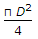B.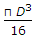C.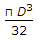D.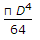5.

A body is subjected to two normal stresses 20 kN/m2 (tensile) and 10 kN/m2 (compressive) acting perpendicular to each other. The maximum shear stress is

A.
 5 kN/m2B.
 10 kN/m2C.
 15 kN/m2D.
 20 kN/m26.

When the shear force diagram is a parabolic curve between two points, it indicates that there is a

A.
 point load at the two pointsB.
 no loading between the two pointsC.
 uniformly distributed load between the two pointsD.
 uniformly varying load between the two points7.

The design of thin cylindrical shells is based on

A.
 hoop stressB.
 longitudinal stressC.
 arithmetic mean of the hoop and the longitudinal stressD.
 geometric mean of the hoop and longitudinal stress8.

The volumetric strain is the ratio of the

A.
 original thickness to the change in thicknessB.
 change in thickness to the original thicknessC.
 original volume to the change in volumeD.
 change in volume to the original volume9.

The torque transmitted by a hollow shaft of outer diameter (D) and inner diameter (d) is

A.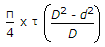B.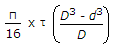C.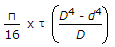D.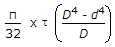10.

The maximum shear stress in a thin cylindrical shell subjected to internal pressure p is

A.
 pd/tB.
 pd/2tC.
 pd/4tD.
 pd/8t11.

The strength of a riveted joint is equal to the

A.
 pull required to tear off the plate per pitch length (Pt)B.
 pull required to shear off the rivet per pitch length (Ps)C.
 pull required to crush the rivet per pitch length (Pc)D.
 minimum value of Pt, Ps or Pc12.

The values of equivalent length (L) and actual length (l) of a column for both ends hinged is the same

A.
 YesB.
 No13.

The assumption, generally, made in the theory of simple bending is that

A.
 the beam material is perfectly homogenous and isotropicB.
 the beam material is stressed within its elastic limitC.
 the plane sections before bending remain plane after bendingD.
 all of the above14.

In a stress-strain diagram for mild steel, as shown in the below figure, the point A represents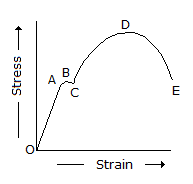A.
 elastic limitB.
 upper yield-pointC.
 lower yield pointD.
 breaking point15.

The centre to centre distance, between two consecutive rivets in a row, is called

A.
 marginB.
 pitchC.
 back pitchD.
 diagonal pitch16.

When a load on the free end of a cantilever beam is increased, failure will occur

A.
 at the free endB.
 at the fixed endC.
 in the middle of the beamD.
 at a distance 2l/3 from free end17.

A simply supported beam 'A' of length l, breadth b, and depth d carries a central point load W. Another beam 'B' has the same length and depth but its breadth is doubled. The deflection of beam 'B' will be __________ as compared to beam 'A'.

A.
 one-fourthB.
 one-halfC.
 doubleD.
 four times18.

The critical neutral axis of a reinforced cement concrete beam is based on the principle that the neutral axis is situated at the centre of gravity of a given section.

A.
 TrueB.
 False19.

For a beam, as shown in the below figure, the deflection at C is (where E = Young's modulus for the beam material, and I = Moment of inertia of the beam section. )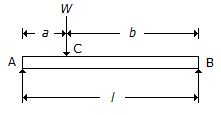A.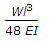B.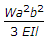C.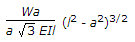D.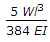20.

The shear modulus of most materials with respect to the modulus of elasticity is

A.
 equal to halfB.
 less than halfC.
 more than halfD.
 none of these